## Indicators

•Cited by SciELO
•Access statistics

•Cited by Google
•Similars in SciELO
•Similars in Google

## Print version ISSN 0120-5609

### Ing. Investig. vol.35 no.3 Bogotá Sept./Dec. 2015

#### http://dx.doi.org/10.15446/ing.investig.v35n3.51676

New heuristic algorithm for dynamic traffic in WDM optical networks

Nuevo algoritmo heurístico para tráfico dinámico en redes ópticas WDM

A. Rodríguez-García1, L. Ramírez-López2, and J.C. Travieso-Torres3

1 A. Rodríguez García: Electrical Engineer. Universidad Nacional de Ingeniería, Peru. ScD in Engineering, Master in Telecommunications. Full-time Professor, Universidad de Santiago de Chile.
E-mail: arturo.rodriguez@usach.cl
2 L. Ramírez López: Electrical Engineer, Master in Systems Engineering, Universidad Nacional de Colombia. PhD in Biomedical Engineering, Universidade de Mogi das Cruzes, Brasil. Associated Professor in Telecommunications En­gineering, School of Engineering, Universidad Militar Nueva Granada.
E-mail: leonardo.ramirez@unimilitar.edu.co
3 J. C. Travieso Torres: Electrical Engineer, Master in Electricity, Instituto Superior Politécnico José Antonio Echeverría, Cuba. ScD in Engineering, Universidad de Santiago de Chile, Chile. Associated Professor, Universidad de Santiago de Chile.
E-mail: juancarlos.travieso@usach.cl

How to cite: Rodríguez-García, A., Ramírez-López, L., & Travieso-Torres, J.C. (2015). New heuristic algorithm for dynamic traffic in WDM optical networks. Ingeniería e Investigación, 35(3), 100-106. DOI: http://dx.doi.org/10.15446/ing.investig.v35n3.51676

ABSTRACT

This paper presents the results from the simulation of Snake One, a new heuristic algorithm, and the comparison made between three heuristic algorithms: Genetic Algorithms, Simulated Annealing, and Tabu Search, using blocking probability and network utilization as standard indicators. The simulation exercise was conducted on WDM NSFNET under dynamic traffic conditions. The results show a substantial decrease of blocking. However, this causes a relative network utilization growth. There are also load intervals which lead to performance improvement, decreasing the number of blocked requests.

Keywords: WDM networks, heuristic algorithm, optical cross connect, dynamic traffic, NSFNET.

RESUMEN

En este artículo se presentan los resultados y la comparación de la simulación de un nuevo algoritmo heurístico llamado Serpiente Uno. La comparación se hace con tres algoritmos heurísticos: Algoritmos Genéticos, Simulated Annealing y Búsqueda Tabú, utili­zando como indicadores estándar la probabilidad de bloqueo y la utilización de la red. La simulación se realizó sobre la WDM NSFNET en condiciones de tráfico dinámicos. Los resultados muestran una disminución sustancial de la probabilidad de bloqueo. Sin embargo, esto causa un aumento relativo de la utilización de la red. También hay intervalos de carga donde el rendimiento mejora, disminuyendo el número de solicitudes bloqueadas.

Palabras clave: Redes WDM, algoritmos heurísticos, conmutadores ópticos, tráfico dinámico, NSFNET.

Received: July 3rd 2015 Accepted: October 15th 2015

Introduction

Since the implementation of data transmission through IPv4, information transport has grown at a substantial rate-mainly due to service generation-increasing data demand. The network was not ready for these services, so it had to evolve to IPv6, and so did the number and quality of services. On the other hand, wireless networks grew to the same or greater extent due to the generation of mobile telephony services, which evolved from 1G to 4G. Meanwhile, transport networks supported the increased load, and routing generated much delay compared to switching, causing transport networks to evolve towards fiber optics. This technology, in turn, evolved into WDM (Wavelength Division Multiplexing) networks. AON (All Optical Network) requires OXC (Optical Cross Connect) commutators. It can commute different wavelengths, where each wavelength develops up to 2.5 Gbps. These networks require establishing lightpaths, which are sets of network links where the data travels along different wavelengths as noted in Zang (2000) and Khan (2005). When networks use different wavelengths along the route, they are called Wavelength Conversion Networks, which have the advantage of making good use of the network, but the disadvantage of increased delay in establishing the connection and higher network implementation cost. Thus, Wavelength Continuity Constraint (WCC) is established. On the other hand, finding a route and its wavelength with WCC to solve this problem is called RWA (Routing Wavelength Assignment), and the state of the art shows different optimization strategies, algorithms and criteria. In general, the route must be found, and then the wavelength is assigned, generating two algorithmic processes. There are other proposals, such as comprehensive solution strategies, which enable finding the route and the wavelength in the same algorithmic process. Additionally, the demand characteristics of these networks vary from static to dynamic, where the former demand context can be optimized, while in latter the problem becomes very complex. Therefore, different algorithmic processes were established, where heuristic algorithms prevail over conventional ones, basically because the conventional ones only find one route, while the heuristic algorithms are able to find several routes more readily. In addition, the latter only look for good routes, while the conventional ones look for the optimum route. The most extensively reviewed algorithms in the state of the art are Genetic as noted in Rodriguez (2008, 2010), Barpanda (2011), Monoyiois (2011) and Rashedi (2012); Particle Swarm Optimization as noted in Rashedi (2012), Artificial Bee Colony in Rashedi (2012), Ant Colony as noted in Zhan (2011), Simulated Annealing as noted in Barpanda (2011), Assis (2010), Hredzak (2011), Na (2011), Rodriguez (2011) and Sakamoto (2013); and Tabu Search as noted in Charbonneau (2010), Boonyopakorn (2011) and Rodriguez (2014); as well as very innovative combinations in Boonyopakorn (2011) and Rodriguez (2014), using different criteria and optimization methods. The TIGUM and GINT research groups in Colombia and Chile propose this new algorithmic processes called Snake One and Snake Two, which will be tested in dynamic demand contexts with an integral strategy, simulating them in the NSFNET Network (National Science Foundation NETwork) as noted in Rashedi (2012). This paper only presents the results of Snake One algorithm, because Snake Two algorithm will be tested later.

Description of the RWA problem.

The purpose of solving the RWA problem is to seek a lightpath (LP) that is not necessarily the optimum one. In an AON a lightpath is defined by a route and a wavelength (λ), and the route is a succession of links (e) in a network.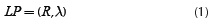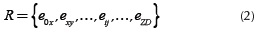Equation (1) represents the pair consisting of a route (R) and the wavelength (λ), where R is the route from the origin (O) to the destination (D) of a set of adjacent links, so that eij represents the link from OXCi to OXCj. Figure 1 represents an optical network with eight nodes numbered 0 to 7, with a link to and from nodes, using three wavelengths represented by dashed 1), solid 2) and dotted 3) lines. The bold lines indicate that they are in use.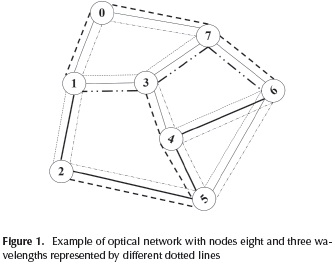In this way we can see routes that go from node 1 to node 6 through different lightpaths, like {e10, e07, e76, λ1}, {e13, e37, e76, λ3}, {e12, e25, e54, e46, λ2}, and from node 2 to node 3 {e25, e54, e43, λ1 }. Assuming that a request arrives at node 3 with node 5 as its destination, we see that there are three ways to arrive at node 5: through node 2, 4 or 6. Through node 2 only one lightpath can be established with λ3; from node 4 only with λ3 , and from node 6 with λ1, λ2 and λ3 .

Depending on the condition, if we choose a route like {e31, e12, e25} the link e31 has λ1 and λ2, link e12 has λ1 and λ3, and link e25 only has λ3. Therefore, there is no way to establish a lightpath with the same wavelength. So to solve the RWA problem we must establish lightpaths that fulfill the WCC without conversion.

State of the art of the RWA.

In the literature there are many innovative solutions for the RWA, but it is achieved distinguish elements that allow understanding the work much better, such as Solution Strategies, Traffic, Optimization Methods and Optimization Criteria.

There are two ways to tackle the RWA problem. One is to divide the problem into a route search and then assigning the wavelength to the route; this solution is the one of the most commonly reviewed ones, because it develops a temporal complexity with less impact, but it requires two algorithmic processes to find the lightpath. On the other hand, there is another way, called comprehensive solution, as noted in Rodriguez (2008), in which the route and the wavelength are obtained through the same algorithmic process, but it has the disadvantage of developing greater temporal complexity. The most widely used indicators are the blocking probability of future demand and network utilization. The aim is to minimize or maximize a criterion such as delay, jitter, etc., which will allow the decrease of blocking probability and of network utilization. Often in WDM the wavelength is increased, which considerably reduces blocking probability, but not necessarily the use of the network. On the other hand, different algorithms that can improve these indicators are tested, but temporal complexity has to be lowered because it is an important factor in increasing blocking probability. On the other hand, network traffic is important to determine if optimization or only finding good routes is possible.

The RWA problem is called SLE (Static Lightpath Establishment) if traffic is static, and DLE (Dynamic Lightpath Establishment) if the traffic is dynamic, as noted in Khan (2005), Rodriguez (2008) and Zang (2001). Traffic is dynamic when the requested average connection time (tC) is less than the average time between arrivals (tLL) of the request for service, as shown in Figure 2.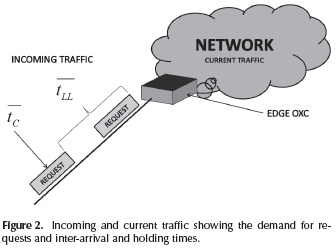In the currently as well as in the future the traffic is dynamic and new solutions will be tested to improve the efficiency of the optical network. Optimization algorithms can be classified in two categories: routing algorithms and wavelength assignment algorithms. Routing algorithms can, in turn, be classified into conventional and heuristic categories: conventional algorithms are those that optimize the route, and therefore, they are used in SLE contexts, which include Dijkstra, Bellman Ford, Floyd Warshall, among others. On the other hand, heuristic algorithms are used to find only good routes; therefore, they are studied in DLE contexts, such as Genetic, Simulated Annealing, Tabu Search, Ant System, and other algorithms. Furthermore, wavelength assignment algorithms are based on simple methods that enable assigning wavelengths rapidly to the links of the route. The most widely used are First Fit (FF), which assigns the first wavelength that fits; Best Fit (BF), which assigns the wavelength that fits best; Next Fit (NF), which tests the next wavelength after the last one that was assigned; Worst Fit (WF), which tests the wavelength under the criterion of maximum network utilization (it does not improve the use of the network. However, it increases blocking probability); Random Fit (RF), which tests the wavelengths randomly; Least Used (LU), which tests the least used one; and Most Used (MU) which tests the most used one as noted in Zang (2001). Finally, regarding the criteria used for optimizing, we can find research based on minimizing or maximizing some criterion such as ASE noise, total congestion, congestion at a link, jitter, total delay, blocking probability, network utilization, congestion of a specific commutator, and others. In general, the work is based on finding the lightpath to satisfy the demand, in order to guarantee the service of future demand and to fit the maximum network availability as possible.

Simulation Exercise Context

Optical networks have evolved into what is called,WDM (Wavelength Division Multiplexing) networks, which allow different wavelength traffic on the same fiber, commonly seen in the single-mode low dispersion type, which enables multiplexing without the appearance of nonlinear phenomena. The most important WDM are NSFNET (National Science Foundation NETwork) as noted in Zang (2001), ARPANET (Advanced Research Project Agency NETwork) as noted in Khan (2005), EON (European Optical Network), UNIT (United Kingdom NETwork), among others. In this research, the NSFNET network is used, simulated without wavelength conversion. Networks with wavelength conversion allow more efficient use of the network's resources than networks without wavelength conversion, but the former have greater delay than the latter. Figure 3 shows the network used in this research (NSFNET), with 14 nodes and 8 wavelengths, without wavelength conversion as noted in Rodriguez (2014).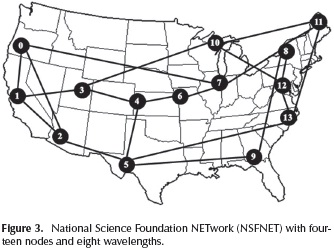The parameters used were similar to those presented in Zang (2001), and comparisons were made of the relevant indicators, such as the probability of blocking and using the network, varying the load in the [0,180] interval with increases of 5 erlangs.

The number of connections made during the simulation exercise in each and every one of the contexts, including Simulated Annealing (SA), Genetic Algorithms (AG), Tabu Search, and Snake-One (SNK1), was 108 connection requests. The non-heuristic algorithm used as reference is the one shown in Zang (2001), which made 104 requests and loads of up to 160 erlangs.

New Snake One Algorithm

This heuristic algorithm simulates the movement of a snake in the cost matrix of the network, with horizontal and vertical movements within a matrix, building the path until it reaches its destination. For this purpose, requests are established to arrive (with Poisson distribution) to the border nodes (Edge OXC), bringing with them three parameters that must be satisfied, otherwise the request must be blocked (Equation (3)):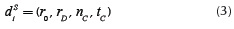Where dsi is the vector that represents the Ith request reaching the sth border node, rD is the identification number of the start node of the demand input, nc is the identification number of the destination node of the demand input, nC is the number of requested connections, and tC is the requested connection time for the (rO , rD) pair. If N is the number of nodes in the network, nCX is the maximum number of connections at each link, and nW is the number of wavelengths at each link (assumed to be equal in all the network's links).

Five matrices have been established: the links matrix and the cost matrix (E, C, Equation (4) and Equation (5)), the Lambda matrix and the time matrix (λ, T, Equation (6) and Equation (7)), and the Snake matrix (S, Equation (8)). Matrix E will retain the network topology, in this case the NSFNET. However, any network you wish to simulate can be configured. The definitions of the matrices are given below. Two more columns were added in matrix in order to save partial calculations like total cost of the rows and the node's label.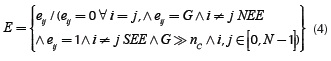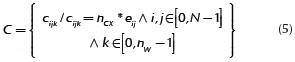SEE = There is a link
NEE = There is no link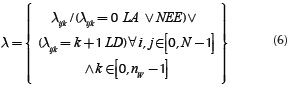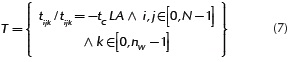LA= Lambda activated
LD= Lambda deactivated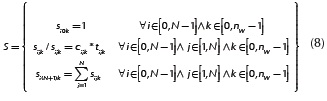The cost matrix C is constructed from matrix links E and NCX. The λ matrix controls the used and the available wavelengths. The T matrix controls the connection time to each request and its elements are: less than zero, when in use and counting until the connection time runs out; zero, when the connection time runs out; and one when the wavelength on the link is available. The S matrix is where the action of Snake One algorithm takes place. The elements of S are positive when the elements of the T matrix are one, i.e. they are available. Other cases will be negative, which means that they will not be taken into account for the horizontal and vertical displacements.

Figure 4 shows the procedures that are carried out to obtain the LP in a single algorithmic process. For example, the seventh request of the demand at node 1 is denoted by d71 = (1,4,1,500) in a network of 8 nodes (N = 8) and 3 wavelengths, i.e., its destination is node 6 and it requests one connection for a time of 500 ms. Then, the Snake matrix is established as a function of the cost matrix, adding two columns: the first one for the labels of the nodes, and the other one for the sum of the cost (Equation(8)), where the required LP will be resolved.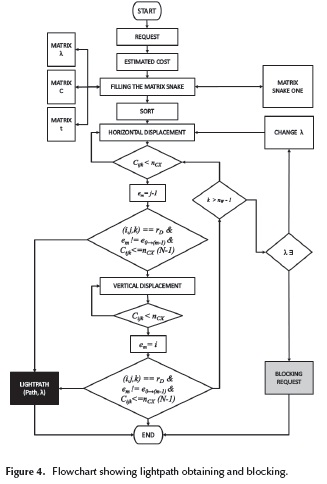The Snake One algorithm will be applied to the matrix of Figure 5 and a route will be sought. If it is not found, a route will be sought in the next wavelength (FF), and if a route is not found in the available wavelengths, the request is blocked. The time matrix is supposed to determine the total availability, that is, t.ijk = 1.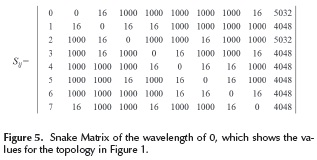For the request be, d71 = (1,4,1,500), Matrix S is arranged in decreasing order according to the cost column. Looking at column 0 of matrix S (Nodes), we locate the row corresponding to the start node 1 (0,0), as seen in Figure 6. Then we move along horizontally until we locate the first cost greater than nC and less than 1000, (value enabling differentiation of a non-existing link) which is located at node 0 (0,1).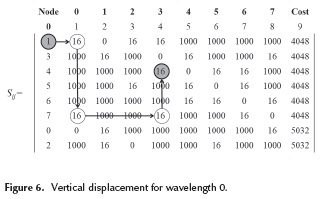Since node 0 is not the destination, we move vertically until we find a cost greater than 0 and less than 1000, which is located at node 7 (5,1). Since node 7 is not the destination, we move horizontally again, locating node 3 (5,4), and then we move vertically locating destination node 4 (2,4), ending the algorithm (Figure 6) because it is the destination proposed in the input request. Therefore, the displacement in matrix S is:

(0,0)→(0,1)→(5,1)→(5,4)→(2,4)

This corresponds to the lightpath 1-0-7-3-4, with wavelength 0, as seen in Figure 7.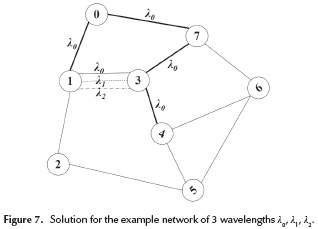Comparison of Results

The simulation exercise was carried out under dynamic traffic conditions, and the heuristic algorithms were subjected to a load variation of 0 to 180 erlangs. It should be stressed that the referential work found in Hui et al. (2001) only simulated up to 160 erlangs, but this does not undermine the comparison. The performance of the proposed Snake One algorithm is important from the standpoint of the blocking probability.

Table 1 presents the heuristics and the average values of the blocking probability using a non-heuristic referential (RF) element from the work shown in Zang (2001). The average value of the Snake One algorithm is 0.32, which is the smallest of all. However, observing the use of the network, the algorithm cannot decrease it, and it increases to an average value of 68.22 %, which is the largest of all the heuristics, as seen in Figures 8 and 9.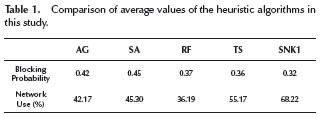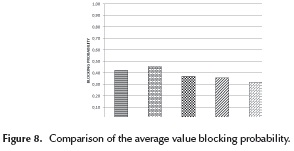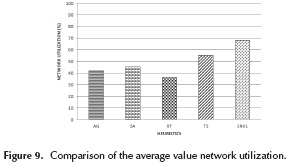When the performance of the blocking probability in the load distribution is examined (Figure 10), the Snake algorithm achieves better performance of up to 130 erlangs with respect to the reference, and up to 140 erlangs in relation to the other heuristics. However, the network utilization (Figure 11) shows no improvement at any load interval, and it appears as the heuristic with the greatest usage of the network's resources.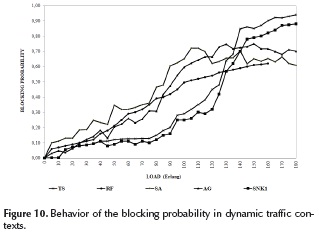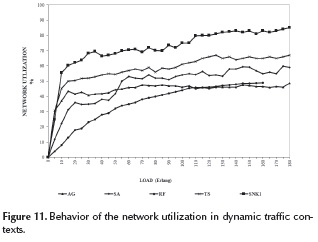Conclusions

The SNK1 algorithm achieves good performance in satisfying the demand, but its performance in network utilization is not acceptable, mainly because its search is made only from the standpoint of the cost matrix, without taking into account the traffic. The next step will be to improve the performance by modifying the algorithm so that it will concentrate the traffic in specific sectors (SNK2). On the other hand, the algorithm responds well up to 130 erlangs, making it recommendable in cases of dynamic high load traffic. Further research on new algorithms and strategies to enhance performance of the indicators is important.

Acknowledgements

Special appreciation is expressed to the Universidad de Santiago de Chile DICYT Program, USACH (Project DICYT N° 061572RG), for its important support in research and development, and particularly to the New Technologies Research Group (GINT-DTI-USACH).

References

Zang H., Jue J. and Mukherjee B. (2000). A Review of Routing and Wavelength Assignment Approaches for wavelength-routed optical WDM networks. Optical Network Magazzine, 1, 47-60.         [ Links ]

Khan A. and Thompson D. (2005) Solving the WDM network operation problem using dynamic synchronous parallel simulated annealing. IEEE SoutheastCon Proceedings, 296­301. DOI: 10.1109/secon.2005.1423262.         [ Links ]

Rodríguez A., Saavedra F. and Ramírez L. (2008) Solución Simultánea del Enrutamiento y asignación de longitud de onda en redes WDM con algoritmos genéticos. IEEE COLCOM 2008, Colombia.         [ Links ]

Rodríguez A. and Saavedra F. (2010) Optimización del Algoritmo Genético para la Solución Integral de Enrutamiento en Redes Fotónicas. Inf. tecnol., 21, 125-133.         [ Links ]

Barpanda R., Turuk A., Sahoo B. and Majhi B. (2011) Genetic algorithm techniques to solve routing and wavelength assignment problem in wavelength division multiplexing all-optical networks. Communication Systems and Networks (COMSNETS), 1, 1-8. DOI: 10.1109/comsnets.2011.5716507.         [ Links ]

Monoyios D. and Vlachos K. (2011) Multiobjective Genetic Algorithms for Solving the Impairment-Aware Routing and Wavelength Assignment Problem. Optical Communications and Networking, 3, 40-47. DOI: 10.1364/JOCN.3.000040.         [ Links ]

Rashedi A., Kavian Y. and Mahani A. (2012) Strobel O. Evolutionary algorithms for solving routing and wavelength assignment problem in optical networks: A comparative study. Transparent Optical Networks, 1, 1-4.         [ Links ]

Barpanda R., Sahoo B., Turuk A. and Majhi B. (2010) Solving large problem instances of the RWA problem using genetic algorithms. Industrial and Information Systems (ICIIS), 1, 41-46. Doi: 10.1109/iciinfs.2010.5578737.         [ Links ]

Zhang-liang W. and Yue-guang L. (2011) An ant colony algorithm with tabu search and its application. Intelligent Computation Technology and Automation (ICICTA), 1, 412-416. DOI: 10.1109/icicta.201 1.387.         [ Links ]

Assis K., Ferreira D. and Giozza W. (2010) Hybrid Algorithms for Routing and Assignment Wavelengths in Optical Networks. Latin America Transactions, 8, 214-220. DOI: 10.1109/TLA.2010.5538395.         [ Links ]

Hredzak B. and Diessel O. (2011) Optimization of placement of dynamic network-on-chip cores using simulated annealing. IECON 2011 IEEE,1, 2400-2405. DOI: 10.1109/ IECON.2011.6119685.         [ Links ]

Na L., Haixing L. and Luo Y. (2011) A new MPLS fault restoration algorithm based on simulated annealing and tabu search. Wireless Communications, Networking and Mobile Computing (WiCOM), 1, 1-4.         [ Links ]

Rodriguez A., Saavedra F. and Ramirez L. (2011) Simulated annealing a proposed solution to the RWA problem in photonic networks. IEEE INTERCON, 2011.         [ Links ]

Sakamoto S., Oda T., Kulla E., Ikeda M., Barolli L. and Xhafa F. (2013) Performance analysis of WMNs using simulated annealing algorithm for different temperature values. Complex, Intelligent, and Software Intensive Systems (CISIS), 1,164-168. DOI: 10.1109/cisis.2013.34.         [ Links ]

Charbonneau N. and Vokkarane V. (2010) Tabu search meta-heuristic for static manycast routing and wavelength assignment over wavelength-routed optical WDM networks. Communications (ICC), 1, 1-5. DOI: 10.1109/icc.2010.5502241.         [ Links ]

Boonyopakorn P. and Meesad P. (2011) A hybrid GA and tabu search approach to find optimal node placement in IP networks. Wireless Communications, Networking and Mobile Computing (WiCOM), 1, 1-4. DOI: 10.1109/wicom.2011.6040610.         [ Links ]

Khelifi L., Zidi I., Zidi K. and Ghedira K. (2013) A hybrid a|pproach based on multi-objective simulated annealing and tabu search to solve the dynamic dial a ride problem. Advanced Logistics and Transport (ICALT), 1, 227-232. DOI: 10.1109/ICAdLT.2013.6568464.         [ Links ]

Charbonneau N. and Vokkarane V. (2012) Static Routing and Wavelength Assignment for Multicast Advance Reservation in All-Optical Wavelength-Routed WDM Networks. Networking, IEEE/ACM Transactions on 2012, 1, 1-14. DOI: 10.1109/TNET.201 1.2175007.         [ Links ]

Zang H., Jue J., Sahasrabuddhe L. and Mukherjee B. (2001) Dynamic Lightpath Establishment in Wavelength-Routed WDM Networks. IEEE Communications Magazine 2001, 39, 100-108. DOI: 10.1109/35.948897.         [ Links ]

Rodriguez A., Gutierrez A., Rivera L. and Ramirez L. (2014) Ruteo y Asignación de Longitud de Onda: Comparación de Algoritmos Genéticos y Simulated Annealing. Inf. tecnol., 4, 13-18.         [ Links ]

Rodriguez A., Ramirez L., Rivera L. and Gutierrez A. (2014) Routing wavelength assignement: A solution with tabu search in dynamic traffic. Ingeniare. Rev. chil. ing., 4, 495-503.         [ Links ]

Rodríguez A., Ramirez L., Espinoza J., Ampuero L. and Ramírez C. (2014) Enrutamiento y Asignación de Longitud de Onda: Algoritmos Heurísticos bajo tráfico dinámico en redes WDM, INTERCON 2014 XXI Congreso Internacional de Ingeniería Electrónica, Eléctrica y Computación, 1, 265-270.         [ Links ]

Rodriguez A., Gutierrez A., Rivera L. and Ramirez L. (2014) Comparing Genetic Algorithms and Simulated Annealing for dynamic traffic routing. Advanced Computer and Communication Engineering Technology Proceedings of the 1st International Conference on Communication and Computer Engineering, Melaka, Malaysia: Springer.         [ Links ]